# Plus Two Maths Chapter Wise Questions and Answers Chapter 9 Differential Equations

Students can Download Chapter 9 Differential Equations Questions and Answers, Plus Two Maths Chapter Wise Questions and Answers helps you to revise the complete Kerala State Syllabus and score more marks in your examinations.

## Kerala Plus Two Maths Chapter Wise Questions and Answers Chapter 9 Differential Equations

### Plus Two Maths Differential Equations Three Mark Questions and Answers

Question 1.
y = e2x(a + bx), a and b are arbitrary constants.
y = e2x(a + bx) ____(1)
Differentiating with respect to x,
$$\frac{d y}{d x}$$ = e2xb + (a + bx)2e2x
$$\frac{d y}{d x}$$ = 2y + be2x ⇒ $$\frac{d y}{d x}$$ – 2y = be2x ____(2)
Differentiating (2) with respect to x,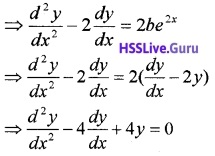Question 2.
y = ex(acosx + 6sinx), a and b are arbitrary constants.
y = ex(acosx + 6sinx) ___(1)
Differentiating with respect to x,
$$\frac{d y}{d x}$$ = ex(-asinx + bcosx) + ex(acosx + bsinx) $$\frac{d y}{d x}$$ = ex(-asin x + b cos x) + y
$$\frac{d y}{d x}$$ – y = ex(-a sin x + b cos x) ____(2)
Differentiating (2) with respec to x,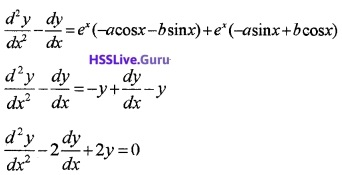Question 3.
y = c1ex + c2 e-x , c1 and c1 are arbitrary constants.
y = c1ex + c2 e-x ___(1)
Differentiating with respect to x,
$$\frac{d y}{d x}$$ = c1ex + c2 e-x __(2)
Differentiating (2) with respect to x,Question 4.
(x – a)2 + 2y2 = a2, a is a arbitrary constants.
(x – a)2 + 2y2 = a2 ___(1)
Differentiating with respect to x,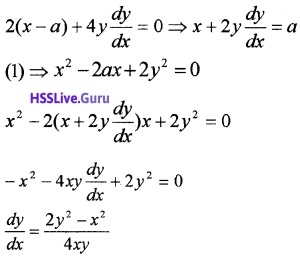Question 5.
Find the equation of a curve passing through the point (0, -2) given that at any point (x, y) on the curve, the product of the slope of its tangent and y coordinate of the point is equal to the x coordinate of the point.
y$$\frac{d y}{d x}$$ = x ⇒ ydy = xdx
Integrating on both sides,
∫ydy = ∫xdx + c
⇒ $$\frac{y^{2}}{2}=\frac{x^{2}}{2}$$ + c ____(1)
Since it passes through (0, -2),Question 6.
Form the DE representing the family of parabolas having a vertex at origin and axis along positive direction of x-axis.
Let (a, 0) be focus of the given family of parabolas.
y2 = 4ax ____(1)
Differentiating with respect to x,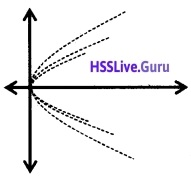Question 7.
For the DE xy $$\frac{d y}{d x}$$ = (x + 2)(y + 2), find the solution curve passing through the point(1,- 1).
xy $$\frac{d y}{d x}$$ = (x + 2)(y + 2)
⇒ $$\frac{y}{y+2} d x=\frac{x+2}{x} d x$$
Integrating on both sides,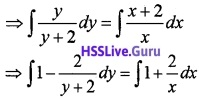⇒ y – 2 log|y + 2| = x + 2log|x| + c ____(1)
Since it passes through (1, -1),
⇒ -1 – 2log|-1 + 2| = 1 + 2log|l| + c
⇒ -2 = c
(1) ⇒ y – 2log|y + 2| = x + 2log|x| – 2.Question 8.
Solve the initial value problem: $$\frac{d y}{d x}$$ = y tan 2x; y(0) = 2.
$$\frac{d y}{d x}$$ = y tan 2x
⇒ $$\frac{d y}{y}$$ tan 2xdx,
This is a variable type
∴∫$$\frac{d y}{y}$$ = ∫tan 2xdx ⇒ log y = $$\frac{1}{2}$$ log|sec 2x| + c
Given y(0) = 2 ⇒ log 2 = $$\frac{1}{2}$$ log|sec 0| + c ⇒ c = log 2
log y = $$\frac{1}{2}$$ log|sec 2x| + log 2 ⇒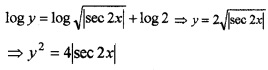### Plus Two Maths Differential Equations Four Mark Questions and Answers

Question 1.
(i) Consider the differential equation given below. (1)
$$\frac{d^{4} y}{d x^{4}}-\sin \left(\frac{d^{3} y}{d x^{3}}\right)=0$$. Write the order and degree of the DE (if defined)
(ii) Find the Differential equation satisfying the family of curves y2 = a(b2 – x2), a and b are arbitrary constants. (3)
(i) 4; degree is not defined

(ii) y2 = a(b2 – x2) ____(1)
Differentiating with respect to x,
2y $$\frac{d y}{d x}$$ = -a2x ⇒ y$$\frac{d y}{d x}$$ = -ax ____(2)
Differentiating (2) with respect to x,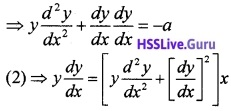Which is the differential equation.Question 2.

1. Find the Differential equation satisfying the family of curves y = ae3x + be-2x, a and b are arbitrary constants. (3)
2. Hence write the degree and order of the DE. (1)

1. y = ae3x + be-2x ____(1)
Differentiating with respect to x,
$$\frac{d y}{d x}$$ = ae3x × 3 + be-2x × -2 ____(2)
Differentiating (2) with respect to x,
⇒ $$\frac{d^{2} y}{d x^{2}}$$ = 9ae3x + 4be-2x ____(3)
Now, (3) + 2 × (2) ⇒ $$\frac{d^{2} y}{d x^{2}}+2 \frac{d y}{d x}=15 a e^{3 x}$$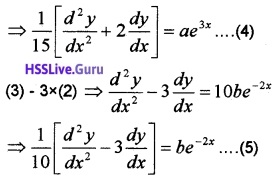Using (4), (5) in (1), we have,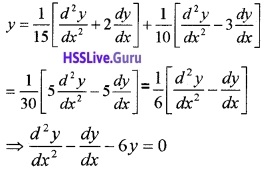2. Order: 2; degree: 1.

Question 3.
Consider the equation of all circles which pass through the origin and whose centres are on the x-axis.

1. Define the general equation of the circle.(1)
2. Find the DE corresponding to the above equation. (3)

1. The general equation of the circle, passing through the origin and whose centers lies on x-axis can be taken as (x – h)2 + y2 = h2 where h being an arbitrary constant.

2. Simplifying (x – h)2 + y2 = h2 we get,
x2 – 2hx + h2 + y2 = h2 ⇒ x2 – 2hx + h2 = 0 _____(1)
Differentiating we get,
2x + 2y $$\frac{d y}{d x}$$ – 2h = 0 ⇒ h = x + y $$\frac{d y}{d x}$$
Substituting in (1) we can eliminate hQuestion 4.
Find a particular solution satisfying the given condition. (x3 + x2 + x +1)$$\frac{d y}{d x}$$ = 2x2 + x, y = 1, when x = 0.Integrating on both sides,
∫dy = ∫$$\frac{2 x^{2}+x}{\left(x^{2}+1\right)(x+1)} d x$$
Splitting into partial fractions we have, (see Unit:7)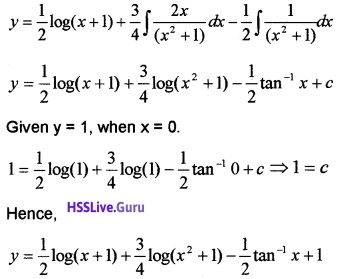Question 5.

1. Write the degree of the DE y’ = 2xy. [0, 1, 2, 3] (1)
2. Express y’ = 2xy in the form Mdx = Ndy. Where M is a function of x and N is the function of y. (2)
3. Solve y’ = 2xy, y(0) = 1 (1)

1. Degree = 1

2. We have, $$\frac{d y}{d x}$$ = 2xy ⇒ $$\frac{d y}{y}$$ = 2xdx, which is of the form Mdx = Ndy.

3. Solution is ∫$$\frac{d y}{y}$$ = 2∫xdx ⇒ log|y| = x2 + c
Given y(0) = 1 ⇒ log|1| = 0 + c ⇒ c = 0
⇒ log|y| = x2 ⇒ y = ex2.

Question 6.
Solve the following DE $$\frac{d y}{d x}=\frac{y^{2}-x^{2}}{2 x y}$$.
$$\frac{d y}{d x}=\frac{y^{2}-x^{2}}{2 x y}$$, this is a Homogeneous DE.
Therefore, put y = vx and $$\frac{d y}{d x}$$ = v + x$$\frac{d v}{d x}$$ to convert it into variable separable form.
The DE becomes,Therefore integrating we get,Question 7.
Solve the linear differential equation $$x \frac{d y}{d x}-y=(x-1) e^{x}$$.
Given, x$$\frac{d y}{d x}$$ – y = (x – 1)ex, dividing both sides by x ,we get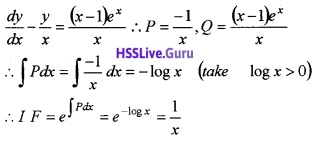Solution is
y × IF = ∫Q(IF)dx + cQuestion 8.
(i) Choose the correct answer from the bracket. The solution of the differential equation xdy + ydx = 0 represents (1)
(a) a rectangular hyperbola
(b) a parabola whose centre is origin
(c) a straight line whose centre is origin
(d) a circle whose centre is origin.
(ii) From the DE of the family of circles touching the x-axis at origin. (3)
(i) (c) a straight line whose centre is origin.

(ii) Let (0, a) be the centre of the circle. Therefore the equation of the circle is
x2 + (y – a)2 = a2
⇒ x2 + y2 = 2ay
⇒ $$\frac{x^{2}+y^{2}}{y}$$ = 2a ____(1)Differentiating with respect to x,Question 9.
Solve the DE x2$$\frac{d y}{d x}$$ = x2 – 2y2 + xy.
x2$$\frac{d y}{d x}$$ = x2 – 2y2 + xy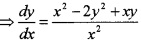this is a Homogeneous DE.
Put y = vx and $$\frac{d y}{d x}=v+x \frac{d v}{d x}$$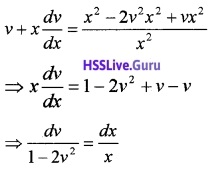Integrating on both sides,Question 10.
Choose the correct answer from the bracket

1. The DE $$\frac{d y}{d x}+\frac{y}{x}$$ = ex, x > 0 is of order …..[0,1,2,3] (1)
2. The integrating factor $$\frac{d y}{d x}+\frac{y}{x}$$ = ex, is……..[x, ex, -x, e-x] (1)
3. Solve $$\frac{d y}{d x}+\frac{y}{x}$$ = ex (2)

1. Order = 1

2. $$\frac{d y}{d x}+\frac{y}{x}$$ = ex is of the form $$\frac{d y}{d x}$$ + Py = Q,
where P = $$\frac{1}{x}$$, Q = ex
IF = e∫Pdx = e∫$$\frac{1}{x}$$dx = elogx = x

3. Solution is y.IF = ∫ex. IFdx
⇒ yx = ∫x.exdx ⇒ yx = x.ex – ∫exdx
⇒ yx = x.ex – ex + c ⇒ yx = ex(x – 1) + c.Question 11.
Solve the DE $$\frac{d y}{d x}=\frac{x+y}{x-y}$$.
$$\frac{d y}{d x}=\frac{x+y}{x-y}$$, this is a Homogeneous DE.
Put y = vx and $$\frac{d y}{d x}$$ = v + x$$\frac{d v}{d x}$$Integrating on both sides,### Plus Two Maths Differential Equations Six Mark Questions and Answers

Question 1.
Consider the DE $$\frac{d y}{d x}=\frac{y^{3}+3 x^{2} y}{x^{3}+3 x y^{2}}$$

1. Identify the DE ? Give reason. (1)
2. Explain the method of solving the DE. (1)
3. Solve the DE. (4)

1. Given DE is a Homogeneous DE. Since y3 + 3x2y and x3 + 3xy2 are Homogeneous functions of same degree (deg = 3).

2. By giving a substitution y = v x and $$\frac{d y}{d x}$$ = v + x$$\frac{d y}{d x}$$
we can convert the DE into variable separable.

3. Now we have, $$\frac{d y}{d x}=\frac{y^{3}+3 x^{2} y}{x^{3}+3 x y^{2}}$$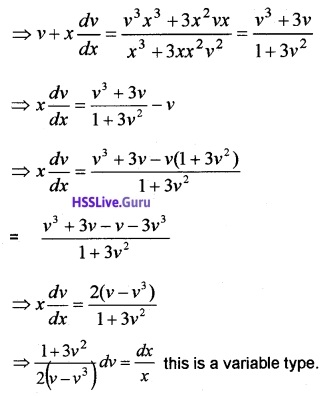∴ Integrating we get,
$$\int \frac{1+3 v^{2}}{2 v\left(1-v^{2}\right)} d v=\int \frac{d x}{x}$$$$\frac{1}{2}$$ log v – log(1 – v2) = log x + log cQuestion 2.
Consider the D.E $$\frac{d y}{d x}+\frac{y}{x}=x^{2}$$

1. Find degree and order of DE . (1)
2. Solve the D.E. (4)
3. Find the particular solution when x = 1, y = 1. (1)

1. Degree: 1, Order: 1.

2. The given D.E is first order linear DE of the form
$$\frac{d y}{d x}$$ + Py = Q. Comparing we get, P = $$\frac{1}{x}$$, Q = x2
∴ ∫Pdx = ∫$$\frac{1}{x}$$dx = logx
Integrating factor (I.F) = e∫Pdx = elogx = x
y.x = ∫x2.xdx + c = ∫x3 dx + c
⇒ y.x = $$\frac{x^{4}}{4}$$ + c ___(1)

3. Given, y = 1 when x = 1, then (1)
⇒ 1 × 1 = $$\frac{1}{4}$$ + c ⇒ c = $$\frac{3}{4}$$
Therefore particular solution is
y.x = $$\frac{x^{4}}{4}$$ + $$\frac{3}{4}$$ ⇒ 4xy = x3 + 3.Question 3.
Consider the equation.$$\frac{d y}{d x}$$ + y = sin x

1. What is the order and degree of this equation? (1)
2. Find the integrating factor. (2)
3. Solve this equation. (3)

1. Order = 1, Degree = 1

2. Given, $$\frac{d y}{d x}$$ + y = sin x is of the form
$$\frac{d y}{d x}$$ + Py = Q ⇒ P = 1, Q = sinx
Integrating factor = e∫Pdx = e∫1dx = ex

3. Therefore solution is
y.IF = ∫Q.IFdx + c ⇒ yex = ∫ex sinxdx + c ____(1)
∫sinx.exdx = ex sinx – ∫cosx.exdx
= ex sin x – cosx.ex – ∫sinx.ex dx
⇒ 2∫ex sin xdx = ex(sin x – cos x)
⇒ ∫ex sinxdx = $$\frac{e^{x}}{2}$$(sinx – cosx)
(1) ⇒ yex = $$\frac{e^{x}}{2}$$(sinx – cosx) + c.Question 4.
Considerthe D.E (x2 – 1)$$\frac{d y}{d x}$$ + 2(x + 2)y = 2(x + 1)

1. Find $$\frac{d y}{d x}$$, degree and order of the above differential equation. (1)
2. Find the integrating factor of the above differential equation. (2)
3. Solve the differential equation. (3)

1. Given, (x2 – 1)$$\frac{d y}{d x}$$ + 2(x + 2)y = 2(x + 1)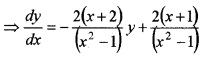Here, Degree = 1, Order = 1.

2. The given DE is of the form $$\frac{d y}{d x}$$ + Py = Q
Where,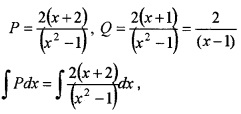Splitting it into partial fractions we get,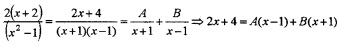Put x = 1, ⇒ 6 = 2B ⇒ B = 3,
put x = -1, ⇒ 2 = -2A ⇒ A = -1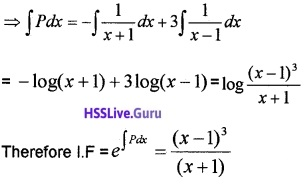3. Solution is y × IF = ∫Q × IFdx + c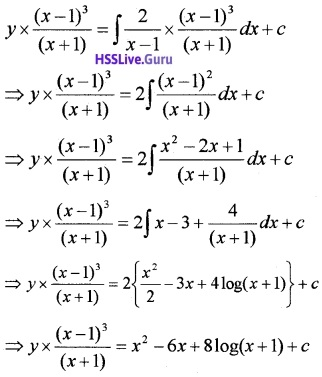Question 5.
(i) The degree of the differential Equation $$\frac{d^{2} y}{d x^{2}}+\cos \left(\frac{d y}{d x}\right)=0$$ is
(a) 2
(b) 1
(c) 0
(d) Not defined
(ii) Solve $$\frac{d y}{d x}$$ + 2y tanx = sinx; y = 0, x = $$\frac{\pi}{3}$$ (5)
(i) (d) Not defined.

(ii) $$\frac{d y}{d x}$$ + 2y tanx = sinx
Then, P = 2tanx, Q = sinx
IF = e∫Pdx = e∫2tanxdx = e2log sec x = sec2 x Solution is; y × IF = ∫Q(IF)dx + c
⇒ ysec2 x = ∫sinx sec2 xdx + c
⇒ ysec2 x = ∫tanx secx dx + c
⇒ ysec2x = secx + c
Here; y = 0, x = $$\frac{\pi}{3}$$
⇒ 0 × sec2 $$\frac{\pi}{3}$$ = sec$$\frac{\pi}{3}$$ + c ⇒ c = -2
⇒ ysec2 x = secx – 2.

Question 6.
(i) The order of the differential equation $$x^{4} \frac{d^{2} y}{d x^{2}}=1+\left(\frac{d y}{d x}\right)^{3}$$ is
(a) 1
(b) 3
(c) 4
(d) 2
(ii) Find the particular solution of the (1 + x2) $$\frac{d y}{d x}$$ + 2 xy = $$\frac{1}{1+x^{2}}$$; y = 0, when x = 1 (5)
(ii) (1 + x2) $$\frac{d y}{d x}$$ + 2 xy = $$\frac{1}{1+x^{2}}$$; y = 0, when x = 1⇒ 0(1 + 12) = tan-11 + c ⇒ c = $$-\frac{\pi}{4}$$
⇒ y(1 + x2) = an-1x – $$\frac{\pi}{4}$$.